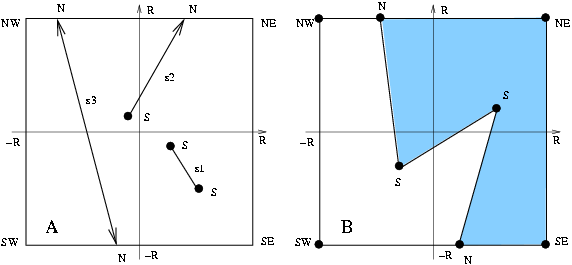CGAL 5.1.3 - 2D Boolean Operations on Nef Polygons
CGAL::Nef_polyhedron_2< T >::Explorer Class Reference

#include <CGAL/Nef_polyhedron_2.h>

## Definition

a decorator to examine the underlying plane map.

An instance E of the data type Explorer is a decorator to explore the structure of the plane map underlying the Nef polyhedron. It inherits all topological adjacency exploration operations from Topological_explorer. Explorer additionally allows one to explore the geometric embedding.

The position of each vertex is given by a so-called extended point, which is either a standard affine point or the tip of a ray touching an infinimaximal square frame centered at the origin. A vertex v is called a standard vertex if its embedding is a standard point and non-standard if its embedding is a non-standard point. By the straightline embedding of their source and target vertices, edges correspond to either affine segments, rays or lines or are part of the bounding frame.Extended geometry: standard vertices are marked by S, non-standard vertices are marked by N. A: The possible embeddings of edges: an affine segment s1, an affine ray s2, an affine line s3. B: A plane map embedded by extended geometry: note that the frame is arbitrarily large, the 6 vertices on the frame are at infinity, the two faces represent a geometrically unbounded area, however they are topologically closed by the frame edges. No standard point can be placed outside the frame.

Creation

Explorer is copy constructable and assignable. An object can be obtained via the Nef_polyhedron_2::explorer() method of Nef_polyhedron_2.

## Types

Iterators, handles, and circulators are inherited from Topological_explorer.

typedef unspecified_type Point
the point type of finite vertices.

typedef unspecified_type Ray
the ray type of vertices on the frame.

## Operations

bool is_standard (Vertex_const_handle v)
returns true iff v's position is a standard point.

Point point (Vertex_const_handle v)
returns the standard point that is the embedding of v. More...

Ray ray (Vertex_const_handle v)
returns the ray defining the non-standard point on the frame. More...

bool is_frame_edge (Halfedge_const_handle e)
returns true iff e is part of the infinimaximal frame.Public Types inherited from CGAL::Nef_polyhedron_2< T >::Topological_explorer
typedef unspecified_type Plane_map
The underlying plane map type.

typedef unspecified_type Point
The point type of vertices.

typedef unspecified_type Mark
All objects (vertices, edges, faces) are attributed by a Mark object.

typedef unspecified_type Size_type
The size type.

typedef unspecified_type Halfedge_around_vertex_const_circulator
circulating the outgoing halfedges in $$A(v)$$.

typedef unspecified_type Halfedge_around_face_const_circulator
circulating the halfedges in the face cycle of a face f.

typedef unspecified_type Hole_const_iterator
iterating all holes of a face f. More...

typedef unspecified_type Isolated_vertex_const_iterator
iterating all isolated vertices of a face f. More...Public Member Functions inherited from CGAL::Nef_polyhedron_2< T >::Topological_explorer
Vertex_const_handle source (Halfedge_const_handle e)
returns the source of e.

Vertex_const_handle target (Halfedge_const_handle e)
returns the target of e.

Halfedge_const_handle twin (Halfedge_const_handle e)
returns the twin of e.

bool is_isolated (Vertex_const_handle v)
returns true iff $$A(v) = \emptyset$$.

Halfedge_const_handle first_out_edge (Vertex_const_handle v)
returns one halfedge with source v. More...

Halfedge_const_handle last_out_edge (Vertex_const_handle v)
returns the halfedge with source v that is the last in the circular iteration before encountering first_out_edge(v) again. More...

returns the edge after e in the cyclic ordered adjacency list of source(e).

returns the edge before e in the cyclic ordered adjacency list of source(e).

Halfedge_const_handle next (Halfedge_const_handle e)
returns the next edge in the face cycle containing e.

Halfedge_const_handle previous (Halfedge_const_handle e)
returns the previous edge in the face cycle containing e.

Face_const_handle face (Halfedge_const_handle e)
returns the face incident to e.

Face_const_handle face (Vertex_const_handle v)
returns the face incident to v. More...

Halfedge_const_handle halfedge (Face_const_handle f)
returns a halfedge in the bounding face cycle of f (Halfedge_const_handle() if there is no bounding face cycle).

Vertex_const_iterator vertices_begin ()
iterator over vertices of the map.

Vertex_const_iterator vertices_end ()
past-the-end iterator for vertices.

Halfedge_const_iterator halfedges_begin ()
iterator over halfedges of the map.

Halfedge_const_iterator halfedges_end ()
past-the-end iterator for halfedges.

Face_const_iterator faces_begin ()
iterator over faces of the map.

Face_const_iterator faces_end ()
past-the-end iterator for faces

Halfedge_around_vertex_const_circulator out_edges (Vertex_const_handle v)
returns a circulator for the cyclic adjacency list of v.

Halfedge_around_face_const_circulator face_cycle (Face_const_handle f)
returns a circulator for the outer face cycle of f.

Hole_const_iterator holes_begin (Face_const_handle f)
returns an iterator for all holes in the interior of f. More...

Hole_const_iterator holes_end (Face_const_handle f)
returns the past-the-end iterator of f.

Isolated_vertex_const_iterator isolated_vertices_begin (Face_const_handle f)
returns an iterator for all isolated vertices in the interior of f.

Isolated_vertex_const_iterator isolated_vertices_end (Face_const_handle f)
returns the past the end iterator of f.

const Pointpoint (Vertex_const_handle v)
returns the embedding of v.

const Markmark (Vertex_const_handle v)
returns the mark of v.

const Markmark (Halfedge_const_handle e)
returns the mark of e.

const Markmark (Face_const_handle f)
returns the mark of f.

Size_type number_of_vertices ()
returns the number of vertices.

Size_type number_of_halfedges ()
returns the number of halfedges.

Size_type number_of_edges ()
returns the number of halfedge pairs.

Size_type number_of_faces ()
returns the number of faces.

Size_type number_of_face_cycles ()
returns the number of face cycles.

Size_type number_of_connected_components ()
calculates the number of connected components of P.

void print_statistics (std::ostream &os=std::cout)
print the statistics of P: the number of vertices, edges, and faces.

void check_integrity_and_topological_planarity (bool faces=true)
checks the link structure and the genus of P.

## ◆ point()

template<typename T>
 Point CGAL::Nef_polyhedron_2< T >::Explorer::point ( Vertex_const_handle v )

returns the standard point that is the embedding of v.

Precondition
E.is_standard(v).

## ◆ ray()

template<typename T>
 Ray CGAL::Nef_polyhedron_2< T >::Explorer::ray ( Vertex_const_handle v )

returns the ray defining the non-standard point on the frame.

Precondition
!E.is_standard(v).